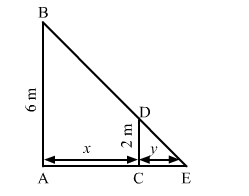# A man 2 metres high walks at a uniform speed of 5 km/hr`
Question:

A man 2 metres high walks at a uniform speed of 5 km/hr away from a lamp-post 6 metres high. Find the rate at which the length of his shadow increases.

Solution:

Let AB be the lamp post. Suppose at any time t, the man CD be at a distance of x km from the lamp post and y m be the length of his shadow CE.Since triangles $A B E$ and $C D E$ are similar,

$\frac{A B}{C D}=\frac{A E}{C E}$

$\Rightarrow \frac{6}{2}=\frac{x+y}{y}$

$\Rightarrow 3 y=x+y$

$\Rightarrow x=2 y$

$\Rightarrow \frac{d x}{d t}=2 \frac{d y}{d t}$

$\Rightarrow \frac{d y}{d t}=\frac{1}{2} \frac{d x}{d t}$

$\Rightarrow \frac{d y}{d t}=\frac{1}{2}(5)\left(\frac{d x}{d t}=5\right)$

$\Rightarrow \frac{d y}{d t}=\frac{5}{2} \mathrm{~km} / \mathrm{hr}$